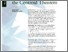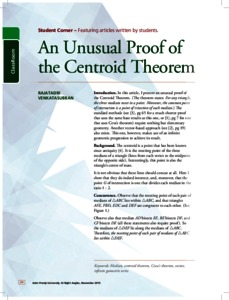# An unusual proof of the centroid theorem

Venkatasubban, Rajatadri (2019) An unusual proof of the centroid theorem. At Right Angles (5). pp. 34-36. ISSN 2582-1873Preview
Text - Published Version

## Abstract

In this article, I present an unusual proof of the Centroid Theorem. (The theorem states: For any triangle, the three medians meet in a point. Moreover, the common point of intersection is a point of trisection of each median.) The standard methods (see , pg 65 for a much shorter proof that uses the same base results as this one, or , pg 7 for one that uses Ceva’s theorem) require nothing but elementary geometry. Another vector-based approach (see , pg 19) also exists. This one, however, makes use of an infinite geometric progression to achieve its result.

Item Type: Articles in APF MagazinesView Item